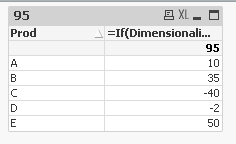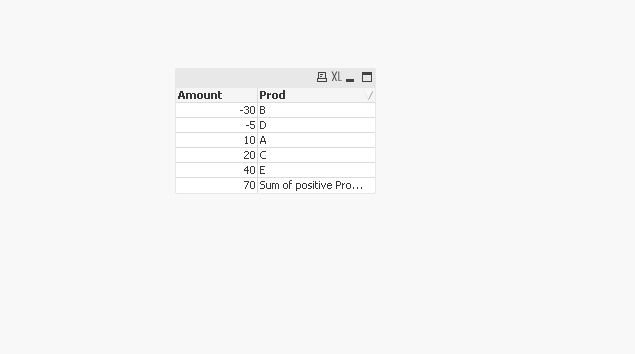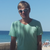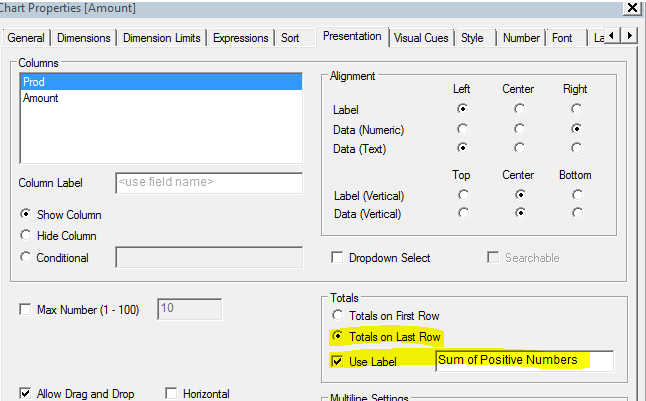# QlikView App Dev

Discussion Board for collaboration related to QlikView App Development.

Announcements
Join this live chat April 6, 10AM EST - QlikView to Qlik Sense REGISTER
cancel
Showing results for
Search instead for
Did you mean:Creator II

## Need logic help

I attached file,

I want to show only the sum of Positive numbers. and in table all number should be present (Positive & Negative).

Thanks,

Lalit kumar

1 Solution

Accepted SolutionsMVP

If in case you have more than one amount value per Prod and you want to check if the Sum(Amount) is greater than 0, then try this

=If(Dimensionality() = 0,Sum({<Prod = {"=Sum(Amount) > 0"}>}Amount), Sum(Amount))7 RepliesPartner

Hi lalit,

try this expression, it will show only positive values..

=if(sum(Amount)>=0,sum(Amount))Specialist

hi,

Please check the attached QVW.

Please mark the question as answered if it meets ur requirement .!Partner

Try this.

Use this expression:

=sum(if(Amount>0,Amount))

I hope this might hekps you

-NagarjunMVP

I don't have license, Can you please check for this on your application whether this is working or not

=sum({<Amount = {'>0'}>} Amount * 1)

Before develop something, think If placed (The Right information | To the right people | At the Right time | In the Right place | With the Right context)Master II

try like this:

=if(sum(Amount)>0,sum(Amount),text(sum(Amount)))Creator III

Hi

Please find the attached app.

Expression:

if( Dimensionality()=0 , sum({<Amount={">0"}>}Amount) , Sum(Amount) )

Few changes in the Presentation Tab,Hope this helps!

Regards

HarishMVP

If in case you have more than one amount value per Prod and you want to check if the Sum(Amount) is greater than 0, then try this

=If(Dimensionality() = 0,Sum({<Prod = {"=Sum(Amount) > 0"}>}Amount), Sum(Amount))